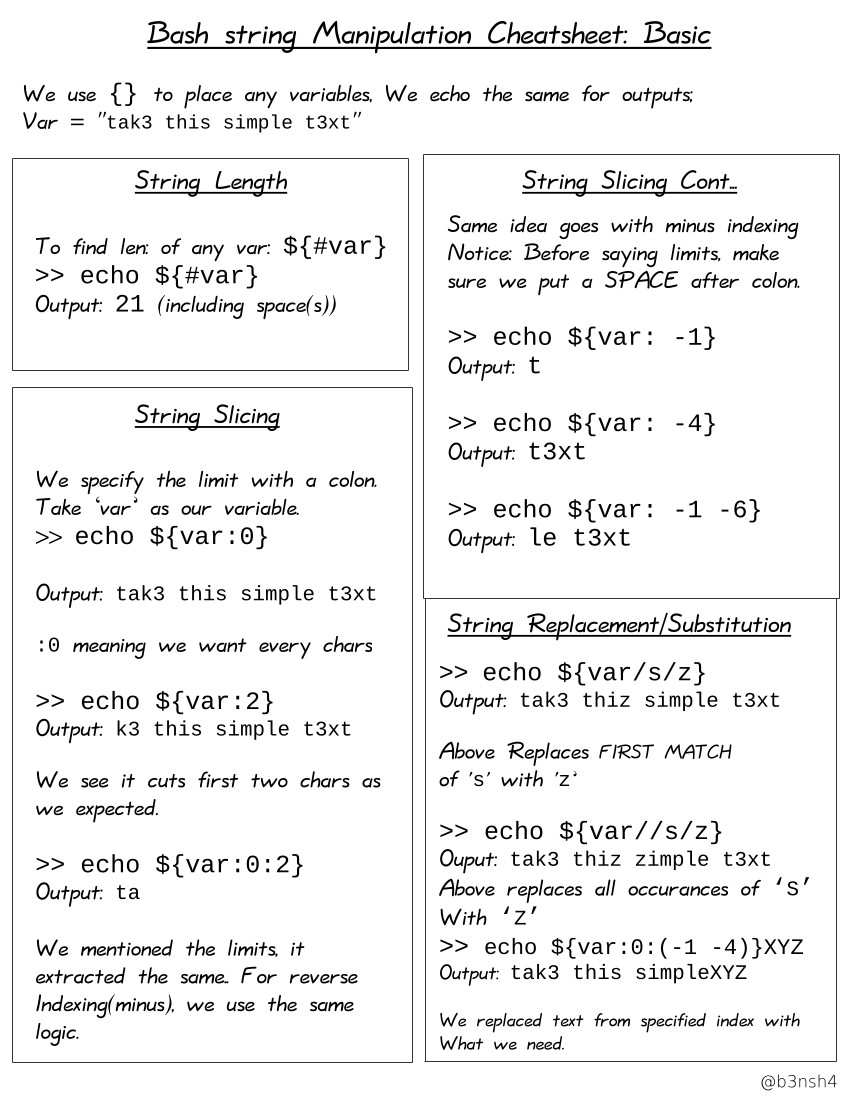# String Manipulation 字串處理

##### 字串長度
``````my_string="abhishek"
echo "length is \${#my_string}"``````

Using expr

``````str="my string"
length=\$(expr length "\$str")
echo "Length of my string is \$length"``````

Using awk

``echo "my string" | awk '{print length}'``

Using wc

``````str="my string"
length=\$(echo -n "my string" | wc -m)
echo "Length of my string is \$length"``````
##### 字串比對

Using wildcards

``````#!/bin/bash

STR='GNU/Linux is an operating system'
SUB='Linux'
if [[ "\$STR" == *"\$SUB"* ]]; then
echo "It's there."
fi``````

Using case

``````#!/bin/bash

STR='GNU/Linux is an operating system'
SUB='Linux'

case \$STR in

*"\$SUB"*)
echo -n "It's there."
;;
esac``````

Using Regex

``````#!/bin/bash

STR='GNU/Linux is an operating system'
SUB='Linux'

if [[ "\$STR" =~ .*"\$SUB".* ]]; then
echo "It's there."
fi``````

Using Grep

``````#!/bin/bash

STR='GNU/Linux is an operating system'
SUB='Linux'

if grep -q "\$SUB" <<< "\$STR"; then
echo "It's there"
fi``````
##### 字串合併
``````str1="hand"
str2="book"
str3=\$str1\$str2``````
##### 字元提取
``````foss="Fedora is a free operating system"
echo \${foss:0:6}``````
``````_admin_ip="202.54.1.33|MUM_VPN_GATEWAY 23.1.2.3|DEL_VPN_GATEWAY 13.1.2.3|SG_VPN_GATEWAY"
do
ufw allow from "\${e%%|*}" to any port 22 proto tcp comment 'Open SSH port for \${e##*|}'
done``````
##### 字元取代
``````foss="Fedora is a free operating system"
echo \${foss/Fedora/Ubuntu}``````
##### Summary: String Manipulation and Expanding Variables
 `\${parameter:-defaultValue}` Get default shell variables value `\${parameter:=defaultValue}` Set default shell variables value `\${parameter:?"Error Message"}` Display an error message if parameter is not set `\${#var}` Find the length of the string `\${var%pattern}` Remove from shortest rear (end) pattern `\${var%%pattern}` Remove from longest rear (end) pattern `\${var:num1:num2}` Substring `\${var#pattern}` Remove from shortest front pattern `\${var##pattern}` Remove from longest front pattern `\${var/pattern/string}` Find and replace (only replace first occurrence) `\${var//pattern/string}` echo "\${PATH//:/\$'\n'}" Find and replace all occurrences `\${!prefix*}` Expands to the names of variables whose names begin with prefix. `\${var,}` `\${var,pattern}` Convert first character to lowercase. `\${var,,}` `\${var,,pattern}` Convert all characters to lowercase. `\${var^}` `\${var^pattern}` Convert first character to uppercase. `\${var^^}` `\${var^^pattern}` Convert all character to uppercase.
##### Cheatsheet: String Manipulation##### String To Integer
``````# \$((string))
sum=\$((3+6))
echo \$sum

a=11
b=3
c=\$((\$a+\$b))
echo \$c``````

Alternate method: expr

Note that it is not a “native” Bash procedure, as you need to have `coreutils` installed (by default on Ubuntu) as a separate package.

``````a=5; b=3; c=2; d=1
expr \$a + \$b \* \$c - \$d``````
##### 去除變數的末端字元 .XXX
``````# Operator "#" means "delete from the left, to the first case of what follows."
x="This is my test string."
echo \${x#* }

is my test string.

# Operator "##" means "delete from the left, to the last case of what follows."
x="This is my test string."
echo \${x##* }

string.

# Operator "%" means "delete from the right, to the first case of what follows."
x="This is my test string."
echo \${x% *}

This is my test

# Operator "%%" means "delete from the right, to the last case of what follows."
x="This is my test string."
\$ echo \${x%% *}

This``````